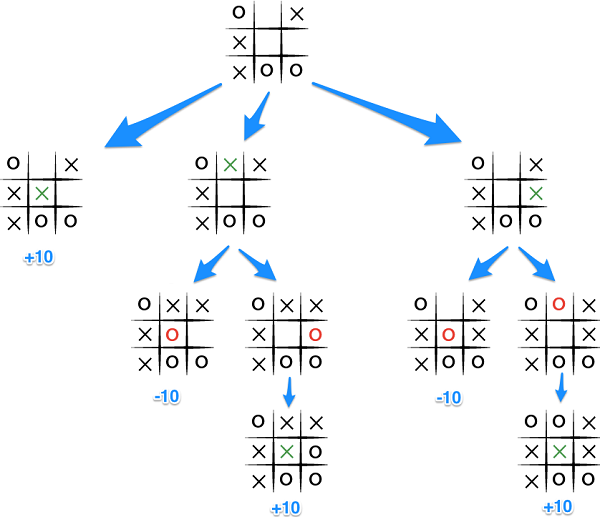## Minimax

Template: Click this link, fork, and invite mrcodeswildride: new project

### Technique

Use a backtracking technique called minimax. For two-player games, minimax is an algorithm that attempts to choose the best possible move for any given game state. It does this by assigning a score to every possible move, and choosing the move with the highest score.
If the move results in an endgame, the score can be computed directly. This is the base case.
If the move doesn't result in an endgame, the score must be computed by looking ahead. This is the recursive case. Minimax assumes that each player will choose the best possible move, so if the opponent is choosing a move, they will choose a move that minimizes the computer's score. If the computer is choosing a move, it will choose a move that maximizes its score.

### Illustration### Pseudocode

```function move():
highestScore = -Infinity

for each possible next move:
score = getScore(nextMove, true)

if score > highestScore:
bestMove = nextMove

function getScore(move, computerTurn):
if game is over:
return score of game state
else:
if computerTurn:
strongestScore = Infinity

for each possible next move:
score = getScore(nextMove, false)
strongestScore = min(score, strongestScore)

return strongestScore
else:
strongestScore = -Infinity

for each possible next move:
score = getScore(nextMove, true)
strongestScore = max(score, strongestScore)

return strongestScore
```